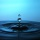Home Communities
IT Knowledge
Inspiration
Languages
EN

# Python - check if string only contains numbers

0 points
Created by:Mark-Rotteveel
477

In this article, we would like to show you how to check if string only contains numbers in Python.

Quick solution:

``````text = "123"
print(text.isdigit())  # True``````
``````text = "123a"
print(text.isdigit())  # False``````

## Practical example

In this example, we use `string.isdigit()` method to check if the strings contain only numbers.

``````numbers = "123"
print(numbers.isdigit())  # True

text = "abc"
print(text.isdigit())  # False

float_number = "1.23"
print(float_number.isdigit())  # False``````

Output:

``````True
False
False``````

Note:

You can also use `isdecimal()` instead of `isdigit()`.

Join to our subscribers to be up to date with content, news and offers.